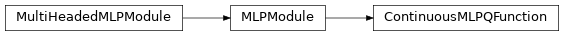# garage.torch.q_functions.continuous_mlp_q_function¶

This modules creates a continuous Q-function network.

class ContinuousMLPQFunction(env_spec, **kwargs)Implements a continuous MLP Q-value network.

It predicts the Q-value for all actions based on the input state. It uses a PyTorch neural network module to fit the function of Q(s, a).

forward(self, observations, actions)

Return Q-value(s).

Parameters
• observations (np.ndarray) – observations.

• actions (np.ndarray) – actions.

Returns

Output value

Return type

torch.Tensor

property output_dim(self)

Return output dimension of network.

Returns

Output dimension of network.

Return type

int